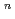To evaluate corrosion behavior of carbon steel, a candidate materials of overpack, buried in soil for a long time, the water pipes buried in freshwater clay for a long time we digged out and the soil environment and the corrosion weight loss of pipes have been researched. From the results, a corrosion model (an empirical equation), an oxygen reduction reaction rate-determing step type, of carbon steel buried in soil was introduced. The corrosion data of under ground pipe collected by the Japan Community Gas Associations was used to increase reliability of the corrosion model equation. These data are one of researches of corrosion behavior of carbon steel buried in soil for a long time studied by at home and abroad. 38 samples buried freshwater clay were selected in 171 samples. With estimating the corrosion velocities and the soil environment factors of the above data, the maximum depth of pit corrosion was calculated by the statistical method of the extreme values using the area of overpack as the recurrent time. The correlation between the soil environment factors and the corrosion weight loss was obtained by the correlation analysis. The corrosion model of the maximum depth of pit corrosion at 0.99 of cumulative probability was compared between the under ground pipe data and the above data. On the reference data and the above data, the corrosion model equation; H = aYwas compared with the maximum depth of pit corrosion at 0.99 cumulative probability. The data of water pipes and community gas pipes at 0.99 cumulative probability showed the reasonable values when these data were compared with the reference data. So that the model was proved as a good corrosion model m the neutral low dissolved oxygen environment.